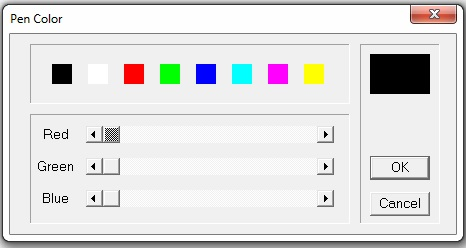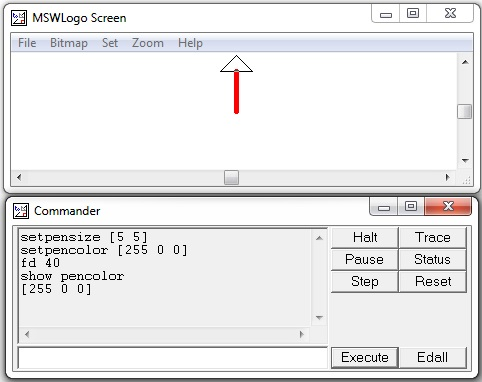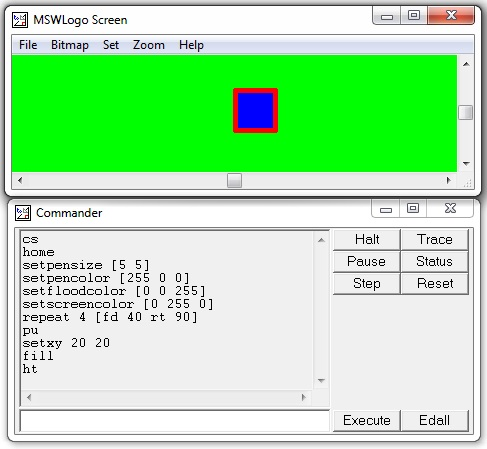# Logo - Color

Computer screens work with red, green and blue components of light, so they are sometimes called RGB screens.

On Logo's Set menu, we can set the color of three screen elements −

• The turtle's pen
• The turtle's fill (like a paint bucket for enclosures)
• The screen backgroundWe set a color by moving these three sliders left and right. Remember that black is the absence of all color and white is all colors together. Mixing light isn't like mixing paint. As an example, if you mix red and green paint, you get a muddy color. Since this is a computer, every color has an internal numeric representation.

On the left end of the sliding scale is zero (0). On the right end is 255, which is kind of like 99 to a computer (It's 28 - 1). Thus black is [0 0 0], red is [255 0 0], green is [0 255 0], blue is [0 0 255]. You can make anything in between these colors, and in all these, there are 256 * 256 * 256 possible colors. That's 28 * 28 * 28, or 24-bits of color — 24-binary digits inside the machine.

The following commands will give you a big fat red pen −

```setpensize [5 5]
setpencolor [255 0 0]
```

When you find a color you like using the sliders, you can ask Logo what it is: choose the pen color, then in the command window, enter the following command.

```show pencolor
```

The following screenshot shows the execution and output of the above code.You can make a colored square using the following steps −

Step 1 − Draw the square with side length 40 using the following command.

```repeat 4 [fd 40 rt 90]
```

Step 2 − Pen up using the following command.

```pu
```

Step 3 − Go to a point inside the square. For example, place the turtle at coordinate (20, 20) using the following command.

```setxy 20 20
```

Step 4 − Fill the square with the set floodcolor. For example, to set the floodcolor to blue use the following command.

```setfloodcolor [0 0 255]
```

The following table lists a few more Color and pen related commands.

Color & Pen command Purpose of the command

setpencolor [ r g b]

setpc [r g b]

Sets the color for turtle’s pen

r g b are numbers in range [0, 255]

setfloodcolor [r g b]

setfc [r g b]

Sets the color for an endorsed area

setscreencolor [r g b]

setsc [r g b]

Sets the color for the background

show pencolor

show floodcolor

show screencolor

Specifies the current values for [r g b] of a named item

Fill

Dumps a bucket of current floodcolor at the cursor’s location

Fill

Dumps a bucket of current floodcolor at the cursor’s location

Try executing the following set of commands −

• cs − To clear the screen.

• home − To bring the turtle at the home place.

• setpensize [5 5] − Setting the pen size.

• setpencolor [255 0 0] − Setting the pen color to red.

• setfloodcolor [0 0 255] − Setting the flood color to blue.

• setscreencolor [0 255 0] − Setting the screen color to green.

• repeat 4 [fd 40 rt 90] − Draw a square with side length 40.

• pu − Pen up.

• setxy 20 20 − Put the turtle at coordinate (20, 20).

• fill − Fill the square with the set floodcolor blue.

• ht − Hide turtle.

You should receive the following output on execution of the above commands.